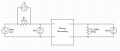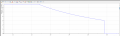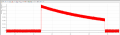# Piezo Energy Harvesting: Power Balance

#### lfelipem

Joined Apr 8, 2019
3
I am doing some piezo and energy harvesting tests, but I am having a hard time calculating the available energy balance. I setup a voltage and current measurement for both the energy harvesting input and output (attached scheme setup).The graphs below demonstrate a test performed, the capacitor voltage is limited to 6.5v and when it reaches 3.5v the output is opened.The main question I need to answer is:

• Is this piezo / harvester set capable of powering a "sersor" that consumes how many Watts for how long? That is, what power do I have available from how long?
Other Questions:

• This measurement setup is correct, should the load / ampere resistor have a smaller value?
• What is the correct method for calculating the energy balance available at the energy harvesting output? Multiplying voltage and current and integrating in time gives me the total energy, right? Dividing energy by time would give me the average power?

Thanks all.

#### Alec_t

Joined Sep 17, 2013
12,735
Welcome to AAC!
the capacitor voltage is limited to 6.5v and when it reaches 3.5v the output is opened.Your schematic doesn't show a capacitor, or any way of controlling its charge/discharge.
should the load / ampere resistor have a smaller value?
We can't tell, as the piezo voltage and the Iin meter sensitivity are unknown, as are the function and characteristics of the magic box called 'Energy harvesting'.
Multiplying voltage and current and integrating in time gives me the total energy, right? Dividing energy by time would give me the average power?
Yes.

#### lfelipem

Joined Apr 8, 2019
3Your schematic doesn't show a capacitor, or any way of controlling its charge/discharge.
The storage capacitor is inside the black box, mounted in the board. Sorry for not making this clear. The energy harvesting datasheet I am using is attached. The storage capacitors seem to be two of 3300uF and 6.5v in parallel (equivalent to 6600uF?!).

We can't tell, as the piezo voltage and the Iin meter sensitivity are unknown, as are the function and characteristics of the magic box called 'Energy harvesting'.
The open circuit piezo generates +-30v. When connected to Energy Harvesting this value is limited to +-8v.

Okay, would that energy be the maximum energy possible to accumulate in capacitors? Is this the total energy available to be used in the best case scenario?
Can you indicate me how, from this energy, I relate to consumption of a particular sensor with power X.

#### Attachments

• 921.9 KB Views: 5

#### Alec_t

Joined Sep 17, 2013
12,735
Okay, would that energy be the maximum energy possible to accumulate in capacitors? Is this the total energy available to be used in the best case scenario?
According to the pdf, the cap voltage is clamped at 6.8V. If the total capacitance is 6600uF then the maximum stored energy is ½ x C x V^2 = 0.5 x 6.6 x 10^-3 x 6.8^2 Joules = 152mJ. You can use stored energy until the cap voltage drops to 3.1V. At that point the stored energy is 0.5 x 6.6 x 10^-3 x 3.1^2 Joules = 32mJ.
Can you indicate me how, from this energy, I relate to consumption of a particular sensor with power X.
If I understand you correctly, you can use 152mJ - 32mJ = 120mJ before the caps needs re-charging. So, for example, a load drawing a constant 1W of power (albeit with the voltage dropping) could, in theory, be powered for 120mS.

#### lfelipem

Joined Apr 8, 2019
3
According to the pdf, the cap voltage is clamped at 6.8V. If the total capacitance is 6600uF then the maximum stored energy is ½ x C x V^2 = 0.5 x 6.6 x 10^-3 x 6.8^2 Joules = 152mJ. You can use stored energy until the cap voltage drops to 3.1V. At that point the stored energy is 0.5 x 6.6 x 10^-3 x 3.1^2 Joules = 32mJ.

If I understand you correctly, you can use 152mJ - 32mJ = 120mJ before the caps needs re-charging. So, for example, a load drawing a constant 1W of power (albeit with the voltage dropping) could, in theory, be powered for 120mS.
Right. Thank you for the informations.

From the initial graphics of the post, integrating the instant power, I obtained the value of 165mJ. I still do not understand why this value is higher than the theoretical, but it is a positive result. From the datasheet, the useful average energy output is well below these values, on the order of 55mJ.

Some additional questions if you can clarify:

- When the capacitor voltage is 6.8v, is it already storing its maximum power capacity? Or if you keep "charging" at this voltage longer can it store more energy?

- How do I calculate the capacitor charging time? Assuming I have 120ms to use on a 1W load. How long could I repeat this use?